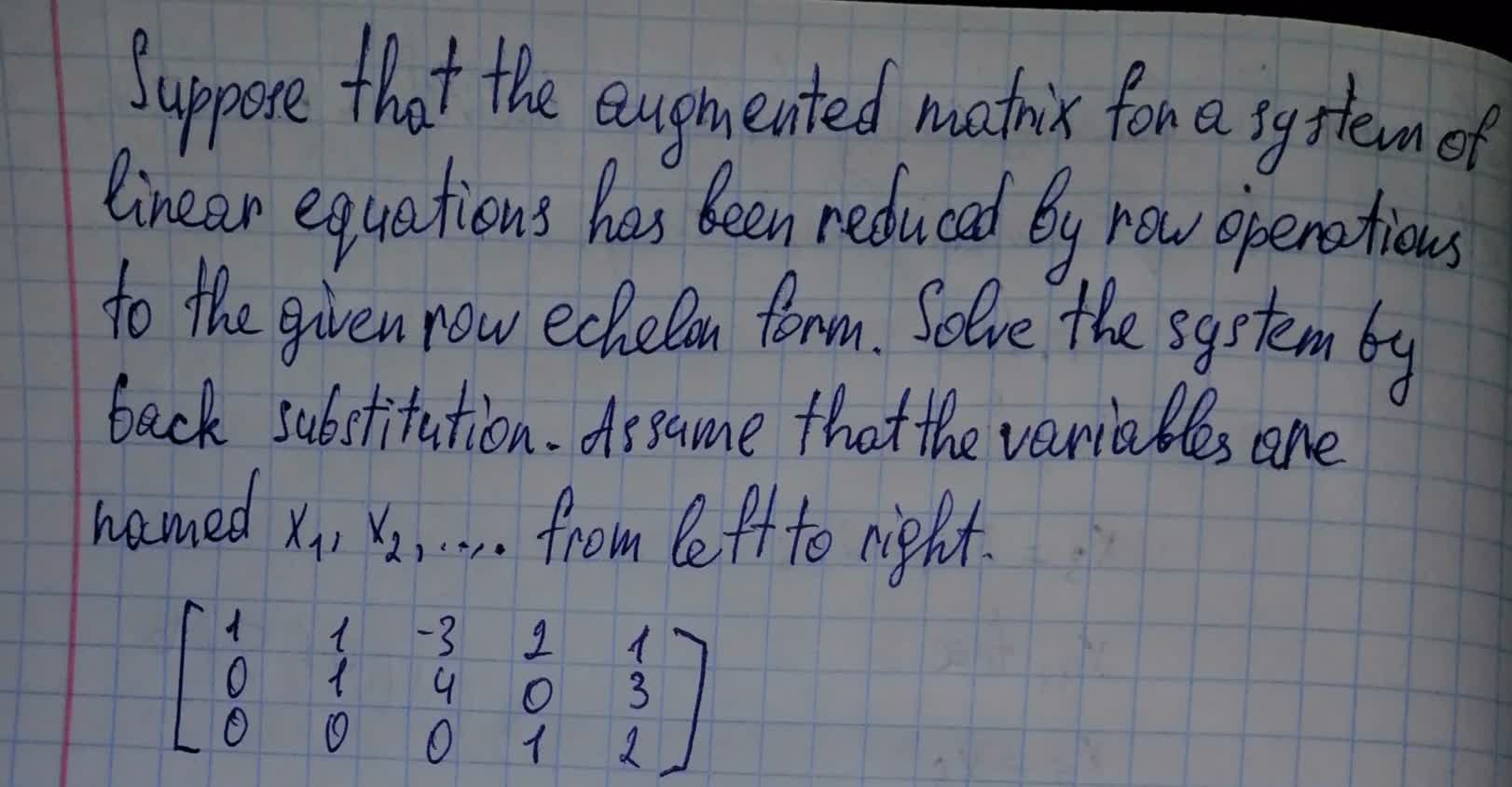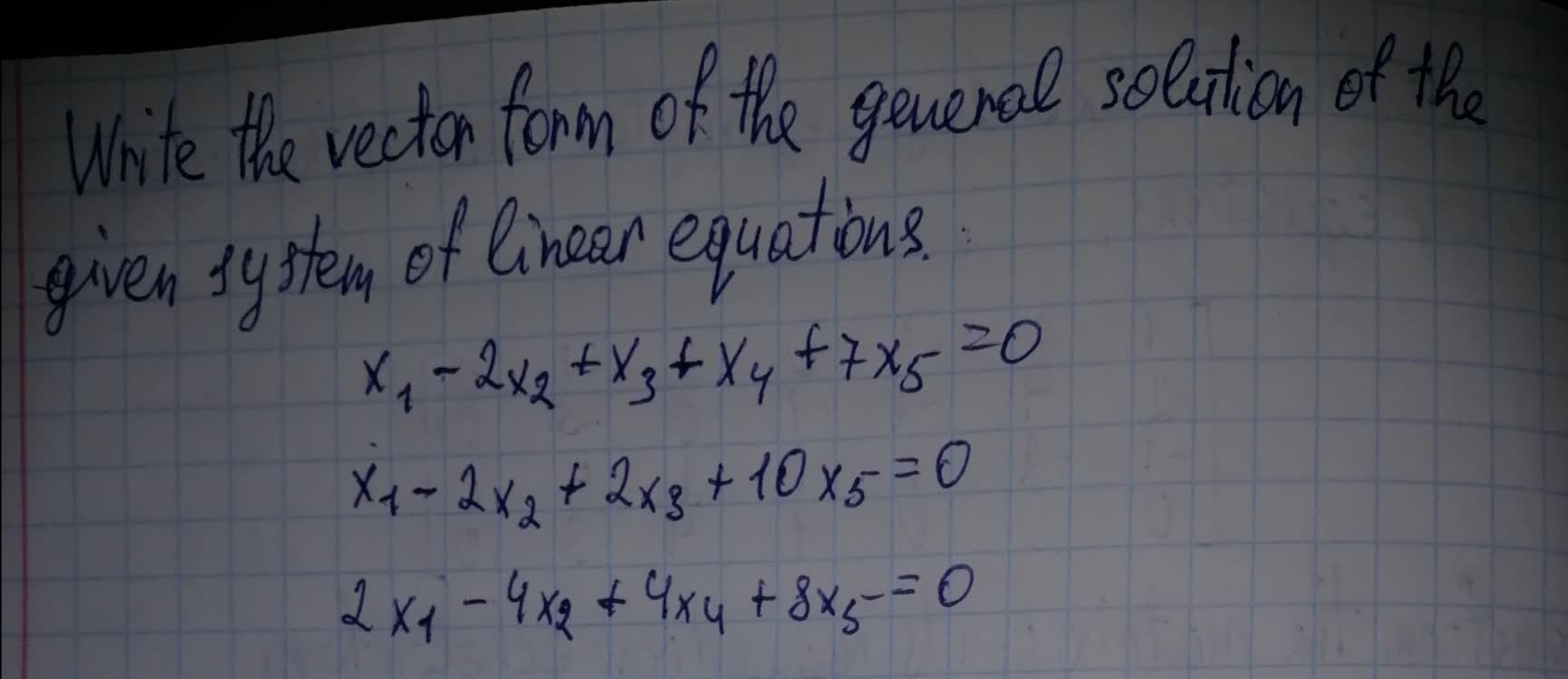# Linear equation forms questions and answersRecent questions in Forms of linear equations
Forms of linear equations
ANSWERED### Suppose that the augmented matrix for a system of linear equations has been reduced by row operations to the given row echelon form. Solve the system by back substitution. Assume that the variables are named $$\displaystyle{x}_{{1}},{x}_{{2}},\ldots$$ from left to right. $$\begin{bmatrix}1 & 1&-3&2&1 \\0&1&4&0&3\\0&0&0&1&2 \end{bmatrix}$$Forms of linear equations
ANSWERED### Write the vector form of the general solution of the given system of linear equations. $$\displaystyle{x}_{{1}}-{2}{x}_{{2}}+{x}_{{3}}+{x}_{{4}}+{7}{x}_{{5}}={0}$$Forms of linear equations
ANSWERED### Suppose that the augmented matrix for a system of linear equations has been reduced by row operations to the given reduced row echelon form. Solve the system. Assume that the variables are named $$x_1, x_2,...x,...$$ ,… from left to right.

Forms of linear equations
ANSWERED### Let $$AX = B$$ be a system of linear equations, where A is an $$m\times nm\times n$$ matrix, X is an n-vector, and $$BB$$ is an m-vector. Assume that there is one solution $$X=X0$$. Show that every solution is of the form $$X0+Y$$, where Y is a solution of the homogeneous system $$AY = 0$$, and conversely any vector of the form $$X0+Y$$ is a solution.

Forms of linear equations
ANSWERED### The reduced row echelon form of a system of linear equations is given.Write the system of equations corresponding to the given matrix. Use x, y; or x, y, z; or $$x_1,x_2,x_3,x_4$$ as variables. Determine whether the system is consistent or inconsistent. If it is consistent, give the solution. $$\left[\begin{array}{cccc|c} 1&0&0&4&2\\0&1&1&3&3\\0&0&0&0&0 \end{array}\right]$$

Forms of linear equations
ANSWERED### The reduced row echelon form of a system of linear equations is given.Write the system of equations corresponding to the given matrix. Use x, y; or x, y, z; or $$x_1,x_2,x_3,x_4$$ as variables. Determine whether the system is consistent or inconsistent. If it is consistent, give the solution. $$\left[\begin{array}{ccc|c}{1} & {0} & {4} & {4} \\ {0} & {1} & {3} & {2} \\ {0} & {0} & {0} & {0}\end{array}\right]$$

Forms of linear equations
ANSWERED### Determine if the statement is true or false, and justify your answer. (a) Different sequence s of row operations can lead to different reduced echelon forms for the same matrix. (b) If a linear system has four equations and seven variables, then it must have infinitely many solutions.

Forms of linear equations
ANSWERED### The row echelon form of a system of linear equations is given. (a)Write the system of equations corresponding to the given matrix.Usex,y;orx,y,z;or $$x_1,x_2,x_3,x_4$$ asvariables. (b)Determine whether the system is consistent or inconsistent. If it is consistent, give the solution. $$\left[\begin{array}{cc|c} 1&2&5\\0&1&-1 \end{array}\right]$$

Forms of linear equations
ANSWERED### Write the vector form of the general solution of the given system of linear equations. x1+4x4=0 x2−2x4 ​

Forms of linear equations
ANSWERED### Write the vector form of the general solution of the given system of linear equations. $$x_1+2x_2−x_3+x_4=0$$

Forms of linear equations
ANSWERED### In the matrix of a system of linear equations, suppose that two of the rows are equal. What can you say about the rowreduced form of the matrix?

Forms of linear equations
ANSWERED### Determine whether the statement is true or false. If the last row of the reduced row echelon form of the augmented matrix of a system of linear equations has only one nonzero entry, then the system is inconsistent.

Forms of linear equations
ANSWERED### $$x+y+2z=3$$ $$3x+2y+2z=4$$ $$x+y+3z=5$$

Forms of linear equations
ANSWERED### Suppose that the augmented matrix for a system of linear equations has been reduced by row operations to the given reduced row echelon form. Solve the system. Assume that the variables are named $$x_1,x_2,...$$ form left to right. $$\begin{bmatrix}1&0&5&3&2\\0&1&-2&4&-7\\0&0&1&1&3\end{bmatrix}$$

Forms of linear equations
ANSWERED### When solving a system of linear equations using matrices, do you prefer to place the augmented matrix in row echelon form or in reduced row echelon form? Give reasons for your choice.

Forms of linear equations
ANSWERED### The reduced row echelon form of the augmented matrix of a system of three linear equations in three variables must be of the form $$\begin{bmatrix}1 & 0 & 0 & a \\ 0 & 1 & 0 & b \\ 0 & 0 & 1 & c \end{bmatrix}$$ where a, b, c, are real numbers. Justify your answer.

Forms of linear equations
ANSWERED### The reduced row echelon form of the augmented matrix of a system of linear equations is given. Tell whether the system has one solution, no solution, or infinitely many solutions. Write the solutions or, if there is no solution, say the system is inconsistent. $$\begin{bmatrix}1 & 0&0&|&-1 \\0 & 1&0&|&3\\0 &0 &1&|&4\\0&0&0&|&0\end{bmatrix}$$

Forms of linear equations
ANSWERED### find an equation of the form $$y=ax^2 + bx + c$$, whose graph passess through the points (2, 3), (-2, 7) and (1, -2). You must use a system of linear equations in three variables for this problem.

Forms of linear equations
ANSWERED### The coefficient matrix for a system of linear differential equations of the form y′=Ay has the given eigenvalues and eigenspace bases. Find the general solution for the system. $$\displaystyleλ_{1}={1}\Rightarrow \left\{\left[\begin{array}{c}2\\ -1\end{array}\right]\right\},λ_{2}={3}\Rightarrow \left\{\left[\begin{array}{c}3\\ 1\end{array}\right]\right\}$$
ANSWERED